# SAS Tutorials: Date-Time Functions and Variables in SAS

Before reading this tutorial, you may wish to review SAS Date Formats and Informats and SAS Date, Time, and Datetime Values.

Dates, times, and date-times are commonly used variable types in research. In SAS, dates and times are considered numeric variables, but they have several special properties to be aware of.

Regardless of how the researcher records dates or times in their dataset, SAS "internally" records datetime variables as integers. This helps to simplify the computations when computing the differences between dates.

• SAS time values are stored internally as the number of seconds between midnight of the current day and another time value.
• SAS datetime values stored internally as the number of seconds between midnight, January 1, 1960, and the specified date and time.
• SAS date values are the stored internally as the number of days between January 1, 1960, and a specified date. Dates after January 1, 1960, are stored as positive numbers; dates before January 1, 1960, are stored as negative numbers.
Table 1: SAS internal values for selected dates.
Date SAS Internal Value
January 1, 1960 0
January 3, 1960 2
December 31, 1959 -1
January 1, 1959 -365
January 1, 1961 366
January 1, 1963 1096

By default, SAS date and time variables are printed using the SAS internal values, rather than a "human-readable" date format. However, dates can be displayed using any chosen format by setting the format in a data step or proc step. (For a full listing of date-time formats, see About SAS Date, Time, and Datetime Values.)

In this tutorial, we show how to compute new variables from dates and times using two major types of date functions: extraction-type functions and computation-type functions.

Date extraction functions are used to extract a portion of a date from a date variable.

• YEAR - Given a number or a variable representing a date or datetime, returns the year.
• QTR - Given a number or a variable representing a date or datetime, returns the quarter.
• MONTH - Given a number or a variable representing a date or datetime, returns the month.
• DAY - Given a number or a variable representing a date or datetime, returns the day (as a number from 1-31).
• WEEKDAY - Given a number or a variable representing a date or datetime, returns the day of the week (as a coded number from 1-7).

Date creation functions construct new date or datetime variables based on their inputs.

• MDY - Given numbers or numeric variables representing the month, day-of-the-month, and year, creates a new date variable.
• INPUT - Given a character variable or value and an appropriate SAS date/date-time informat, convert a character variable to a date variable.
• INTNX - Given a time interval and a starting date, create a new date variable by adding or subtracting the time interval from the starting date.

Date computation (or "date difference") functions carry out arithmetic operations on dates; for example, computing the elapsed time between two dates.

• DATDIF - Given two SAS dates or datetimes, computes the difference between the dates in days.
• YRDIF - Given two SAS dates or datetimes, computes the difference between the dates in years.

Lastly, we also touch on date constants, which are useful if you want to subset a dataset based on date values, or calculate a date variable based on a reference date.

For most of the examples on this page, we use two short, 5-row datasets.

• In the dataset called sample, the main variables are DOB (a date variable representing an individual's date of birth) and Admdate (a date variable representing an individual's admission date to college).
• In the dataset called stringdates, the main variables are datestring1 and datestring2: both are character variables containing date values using different date patterns.

If you'd like to try out any of the examples on this page, you can recreate the sample datasets in your instance of SAS by running this code first:

/*Sample dataset with two date variables*/
DATA sample;
INPUT id DOB :MMDDYY10. Admdate :MMDDYY10.;
DATALINES;
1 08/23/1991 12/08/2013
2 06/07/1994 06/12/2013
3 03/15/1993 11/17/2012
4 12/18/1991 07/19/2013
5 01/03/1991 12/03/2012
;
RUN;

/*Sample dataset with two character variables representing dates*/
DATA stringdates;
INPUT @1 id
@5 datestring1 $17. @25 datestring2$9.;
DATALINES;
1	October 5, 2023		5oct2023
2	January 15, 1989	15jan1989
3	February 29, 2020	29feb2020
4	November 30, 1889	30nov1889
5	April 1, 2001		1apr2001
;
RUN;


After running this code, you should be able to run the example codes in the following sections.

## YEAR Function

Given a SAS date value, the YEAR function extracts the year as a numeric value.

### Syntax

YEAR(date);

Where date is a SAS date value that is specified either as a variable or as a SAS date constant.

### Example

DATA sample;
SET sample;
yr = YEAR(DOB);
RUN;

Here the YEAR function extracts the year portion from the date value variable DOB and saves it in the new numeric variable yr.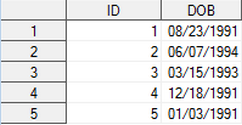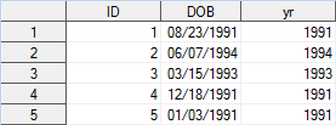## QTR Function

Given a SAS date value, the QTR function extracts the quarter as a numeric value.

### Syntax

QTR(date);

Where date is a SAS date value that is specified either as a variable or as a SAS date constant.

### Example

DATA sample;
SET sample;
quarter = QTR(DOB);
RUN;

Here the QTR function extracts the quarter value from the date value variable DOB and saves it in the new numeric variable quarter.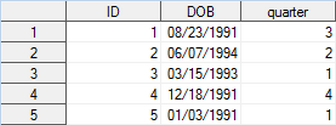## MONTH Function

Given a SAS date value, the MONTH function extracts the month as a numeric value.

### Syntax

MONTH(date);

Where date is a SAS date value that is specified either as a variable or as a SAS date constant.

### Example

DATA sample;
SET sample;
mn = MONTH(DOB);
RUN;

Here the MONTH function extracts the month value from the date variable DOB and saves it in the new numeric variable mn.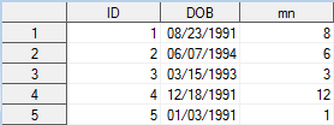## DAY Function

Given a SAS date value, the DAY function extracts the day of the month as a numeric value (between 1-31).

### Syntax

DAY(date);

Where date is a SAS date value that is specified either as a variable or as a SAS date constant.

### Example

DATA sample;
SET sample;
days = DAY(DOB);
RUN;

Here the DAY function extracts the day value from the date variable DOB and saves it in the new numeric variable days.## WEEKDAY Function

Given a SAS date value, the WEEKDAY function extracts the day of the week from a SAS date value as a number from 1-7.

 Value Day of the week 1 Sunday 2 Monday 3 Tuesday 4 Wednesday 5 Thursday 6 Friday 7 Saturday

### Syntax

WEEKDAY(date);

Where date is a SAS date value that is specified either as a variable or as a SAS date constant.

### Example

DATA sample;
SET sample;
wkday = WEEKDAY(DOB);
RUN;

Here the WEEKDAY function extracts the day of the week value from the date variable DOB and saves it in the new numeric variable wkday.## INPUT Function (Converting Character Variables to Dates)

Depending on how your data was imported into SAS, you may find that you have character variables that you want to be recognized as actual dates. Since date variables in SAS are a special type of numeric variable, we can use the INPUT() function, which converts a character variable to a numeric variable according to a specified informat.

### Syntax

INPUT(datestring, informat);

Where datestring is a character variable or character string, and informat is a recognized SAS date/date-time informat specifying how the character string should be parsed. Your choice of informat will depend on the pattern used to record the dates.

### Example

DATA stringdates2;
SET stringdates;
datevar1=INPUT(datestring1, ANYDTDTE40.);
datevar2=INPUT(datestring2, DATE9.);
RUN;

Here, we start with dataset with two different character variables we want to convert to "true" date variables. The same calendar dates are represented in both columns, but they use different encodings. We use the ANYDTDTE40. informat on variable datestring1, which uses the full month name, day, and year; and we use the DATE9. informat on the variable datestring2, which uses the day, abbreviated month, and 4-digit year.Notice that datevar1 and datevar2 do not have a date format applied to them, so we see their numeric representation (see Table 1). this makes it easier to see that after applying the appropriate informats, both character variables resolve to the same numeric values. (If we did not want to see the numeric representation of the dates, we could modify our data step code to add FORMAT statements for datevar1 and datevar2.)

## INTNX Function

Given a valid SAS time interval and a SAS date, increment the date by the time interval and return a date.

### Syntax

INTNX(interval, date, increment);

Where

• interval is a valid SAS time interval (see Table 3)
• date is a SAS date variable or value representing the "initial" date
• increment is an integer or integer-valued numeric variable representing the number of time interval units to adjust by

For the interval argument, time intervals must be specified using a valid character string. In their simplest form, these names can be one of the following:

Table 3: Table of common time interval names for the INTNX function
SAS Interval Names Definition
'hour' One hour interval
'day' Daily interval
'week' Weekly interval, weeks beginning on Sunday (default)
'weekday' Five-day work week with a Saturday-Sunday weekend
'tenday' Ten-day periods
'semimonth' Twice-monthly interval
'month' Monthly interval
'qtr' Quarterly interval
'semiyear' Twice-per-year interval
'year' Yearly interval

You can add the prefix 'dt' to any of the above codes except 'hour' to indicate a date-time interval (e.g. 'dtweek', 'dtmonth').

### Example

DATA sample2;
SET sample;
RUN;

Here, we demonstrate the breadth of the INTNX function:

• admdate_addday is one day after the admission date
• admdate_subday is one day before the admission date
• admdate_addweekday is one "week day" after the admission date (i.e., the next calendar day that is not a Saturday or Sunday)
• admdate_addweek is one week after the admission date (7 calendar days)
• admdate_addmonth is one month after the admission date
• admdate_add6weeks is six weeks after the admission date

## MDY Function

The MDY function creates a new SAS date value, given numeric values representing the month, day, and year.

### Syntax

MDY(month, day, year);

Where

• month is a number from 1-12 (or a numeric variable containing that number);
• day is a number from 1-31 (or a numeric variable containing that number)
• year is a two- or four-digit year (or a numeric variable containing that number)

### Example

#### Sample Data

For this example only, use this dataset as your input dataset:

DATA sample2;
INPUT id days mn yr;
DATALINES;
1 23 8 1991
2 7 6 1994
3 15 3 1993
4 18 12 1991
5 3 1 1991
;
RUN;

#### Syntax

DATA sample2;
SET sample2;
date = MDY(mn, days, yr);
FORMAT date MMDDYY10.;
RUN;

Here a new variable date will be created by combining the values in the variables mn, days, and yr using the MDY function. The (optional) MMDDYY10. format tells SAS to display the date values in the form MM/DD/YYYY.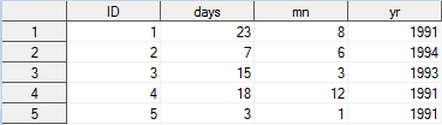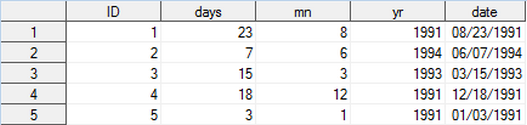## DATDIF Function

Given two SAS dates, function DATDIF returns the number of days between those two dates.

### Syntax

DATDIF(start_date, end_date, basis);

Where

• start_date is a SAS date value that specifies the starting date;
• end_date is a SAS date value that specifies the ending date;
• basis specifies a character constant (see Table 2) that represents how SAS should count the number of days in a month and year.

The basis argument (which is required for both the DATDIF and YRDIF functions) determines how the date arithmetic is carried out. Specifically, it determines the number of days that should be used to characterize the period of time in a month or year. In SAS 9.3, there are five possible options that can be used, which are indicated by the following character strings:

Table 2: Valid character strings for basis argument in DATDIF and YRDIF functions.
Basis Value Meaning
'30/360' Specifies a 30 day month and a 360 day year, regardless of the actual number of calendar days in a given month or year.
'ACT/ACT' or 'Actual' Uses the actual number of days or years between dates.
'ACT/360' Uses the actual number of days in a particular month, and 360 days as the number of days in a year (regardless of the actual number of days in a given year.)
'ACT/365' Uses the actual number of days in a particular month, and 365 days as the number of days in a year (regardless of the actual number of days in a given year.)
'AGE' Valid for YRDIF function only. Specifies that a person's age will be computed. This is the default option for YRDIF.

### Example

DATA sample;
SET sample;
RUN;

Here the DATDIF function returns the difference between two date variables (DOB and Admdate) in number of days and saves it in the new numeric variable date.## YRDIF Function

Given two SAS dates, the YRDIF function returns the number of years between two dates.

### Syntax

YRDIF(start_date, end_date, basis);

Where

• start_date is a SAS date value that specifies the starting date;
• end_date is a SAS date value that specifies the ending date;
• basis specifies a character constant (see Table 2) that represents how SAS should count the number of days in a month and year.

### Example

DATA sample;
SET sample;
RUN;

Here the YRDIF function gives the difference between two dates (DOB and Admdate) in number of years and saves it in the new numeric variable years.When writing a SAS script, there may be times when you need to refer to a single, specific date. In SAS terminology, we would refer to this as a date constant: a reference to a single, specific date. Two scenarios where this may come up are filtering a dataset based on a date variable (i.e., select cases with date values before or after a reference date) and calculating elapsed time between a particular starting date and a date variable.

In SAS, date-time constants must be specified in the pattern DDmmmYYYY. If you attempt to specify a date using any other date pattern (such as MM/DD/YYYY or YYYY-MM-DD), SAS will return an error message. Additionally, these dates must be wrapped in quotation marks and followed by the letter d. For example, in order to represent the date 8/23/1991 as a SAS date constant, it would be written:

'23aug1991'd

Alternatively, date creation functions like MDY() can be used to construct date constants. For example, the date 8/23/1991 could be represented using the syntax

MDY(8, 23, 1991)

Within a data step, a filtering task to retain only cases with a date of birth after 8/23/1991 might look like:

DATA sample_filtered;
SET sample;
IF DOB > '23aug1991'd THEN OUTPUT;
RUN;